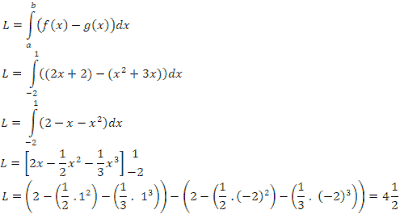-->

# Calculation Of The Area Between The Curve And The X Axis

After you have skillfully calculated certain integrals, below will be presented a calculation of the area between the curve and the X-axis.

Example 7.
Calculate the area bounded by the curve y = x2, X axis, line x = 1, and line x = 2.

So, the area is 2.33 units of area

The size of the area shown in Figure-1 is positive, because the product of f (x) and ∂x is negative.

Because the area is always positive, then,

Example 1:
Calculate the area under the X-axis which is limited by the curve y = 4- 2x, the X-axis, and the line x = 4. Answer:
The shaded area is located below the X-axis, the area

So, the area under the X-axis is limited by the curve y = 4 - 2x, the x-axis and line x = 4 are 4 units of area.

Example 2.

Calculate the area bounded by the curve y = 4x – x2, X axis, line x = 0 and line x = 6

By looking at Figure 3, to calculate the area it needs to be divided into two parts. namely,

Thus, the total area is L1 + L2 = 10 2/3 + 10 2/3 = 21 1/3  unit area

### Area Between Two Curves f(x) and g(x)

Suppose that f and g are continuous functions in [a, b] and f (x) ≥ g (x) in that interval, with the terms y1 = f(x) and y2 = g(x)  not intersecting on [a, b]. Figure-4 shows the above information specifically for the functions of f and g which are non-negative.

In Fig. 4 it appears that the area limited by the curves f and g in [a, b] is

Note: In the same way it can be shown that the formula also applies to negative f and g functions or positive f functions and negative g functions as shown in Figure-5 below,

Example 3.
Calculate the area limited by the curve y = x2 + 3x  and y = 2x + 2.
First, determine the intersection of the two curves, as the boundary and the lower limit.

### x2 + 3x = 2x + 2 x2 + x – 2 = 0 (x + 2)(x - 1) <=> x = -2 or x = 1 So, the boundaries are x = -2 and x = 1, so the area bounded by the two curves is,So, the area is 4 1/2 units of area.